# NCERT Solutions For Class 11 Math Chapter – 7 Exercise – 7.3

NCERT Solutions For Class 11 Math Chapter – 7 Exercise – 7.3

Q1. How many 3 – digit numbers can be formed by using the digits 1 to 9 if no digits are repeated ?

Solution:-

3 – digit numbers have to be formed by using the digits 1 to 9.

Here, the order of the digits matters.

Therefore , there will be as many 3 – digit numbers as there are permutations of 9 different digits taken 3 at a time.

Hence , required number of 3 -digit numbers are

P3 = 9!/(9-3)! = 9!/6! = 9 X 8 X 7 X 6!/6! = 504

Q2. How many 4 – digit numbers are there with n digit repeated?

Solution:-

The thousands place of the 4 – digit number is to filled with any of the digits from 1 to 9 as  the digit 0 cannot be included Therefore, the number of ways in which thousands place can be filled is 9.

The hundreds tens and unit place can be filled by any of the digits from 0 to 9. However , the digits cannot be repeated in the 4 – digits numbers and thousands place in already occupied with a digit. The hundreds tens, and units place is to be filled by the remaining 9 digits.

Therefore, there will be as many such 3 – digits numbers as there are permutations of 9 different  digits taken 3 at a time.

Number of such 3 – digit numbers are

P3 = 9!/(9-3)! = 9!/6! = 9 x 8 x7x6!/6! = 504

Thus , by multiplication principle the required  number of 4 digit number are 9 x 504 = 4536.

Q3. How many  3 – digits even numbers can be made using the digits 1,2,3,4,6,7 If no digit is repeated?

Solution:-

3 – digit even number are to be formed using the given  six – digits  , 1,2,3,4,6 and 7 without repeating the digits.

Then, units can be filled in 3 ways by any of the digits 2,4 or 6.

Since the digits cannot be repeated in the 3 – digit numbers and units place is already occupied with a digit (which is even) the hundreds and tens place is to be filled by the remaining 5 digits.

Therefore, the  number of ways in which hundreds and tens place can be filled with the remaining 5 digits is the permutation of 5 different digit is taken 2 at a time.

Number of ways of filling hundreds and tens place is

P2 = 5!/(5-2)! = 5!/3! = 5 X 4 X 3!/3! = 20

Thus, by multiplication principle , the required number of 3 – digit numbers is 3 x 20 = 60.

Q4. Find the number of 4 – digit numbers that can be formed using the digits 1,2,3,4,5 if no digit is repeated. How many of these will be even?

Solution:-

• digit numbers are to be formed using the digits 1,2,3,4 and 5.

There will  be as many 4 – digit numbers as there are permutations of 5 different digits taken 4 at a time.

Therefore , required number of 4 – digit number are

P4 = 5!/(5-4)! = 5!/4! = 5 X 4 X 3 X 2 X 1 = 120

Among the 4-digit numbers formed by using the digits. 1,2,3,4,5 even numbers end with either 2 or 4.

The number of ways in which units place is filled with digits is 2.

Since the digits are not repeated and the units place is already occupied with a digit ( which is even) the remaining places are to be filled by the remaining 4 digits.

Therefore, the number of ways in which the remaining places can be filled is the permutation of 4 different digits taken 3 at time.

Number of ways of filling the remaining places is

P3 = 4!/(4-3)! = 4!/1! = 4 X 3 X 2 X 1 = 24

Thus , by multiplication principle , the required number of even numbers are 24 x 2 = 48.

Q5.  From a committee of 8 person, in how many ways can we choose a chairman and a vice chairman assuming one person cannot hold more than one position?

Solution:-

From a committee of 8 persons , a chairman and a vice chairman are to be chosen in such a way that one person cannot hold more than one  position.

Here, the number of ways of choosing a chairman and a ice chairman is the permutation of 8 different objects taken 2 at a time.

Thus , required number of ways is

P2 = 8!/(8-2)! = 8!/6! = 8 X 7 X 6!/6! = 56

Q6. Find n if n-1 P3 : NP4 = 1:9

Solution:-

N-1P3 : P4 = 1:9

N-1P3/NP4 = 1/9

N = 9

Q7. Find r if

• 5PR = 26PR-1
• 5PR= 6PR-1

Solution:-

• It is given that 5PR= 26PR-1

= (7-r)(6-r) = 12

42 – 7r – 6r + r2 = 12

r2 – 13r + 42 = 12

r2 – 13r + 42 – 12 = 0

R2 -13r + 30 = 0

R2 – 10r – 3r + 30 = 0

R(r-10) -3(r-10) = 0

(r-3) (r-10) = 0

(r-3) = 0 or (r-10) = 0

R = 3 or r = 10

It is known that, nPR  = N!/(N-R)! where 0 < r < n

Therefore, 0 < r < 5 and r  ≠ 9

Thus , r = 3

• It is given that 5PR= 6PR-1

= 5!/(5-R)! = 6!/(6-R+1)!

= 5!/(5-R)! = 6 X 5!/(7-R)!

= (7-r)(6-r) = 6

= 42 – 7r – 6r + r2 = 6

= r2  -13r + 42 -6 = 0

= r2 -13r + 36 = 0

= r2 -9r – 4r + 36 = 0

= r(r-9) -4(r-9) = 0

= (r-4)(r-9) = 0

= r = 4 or r = 9

It is known  that, nPR = n

8. How many words, with or without meaning, can be formed using all the letters of the word EQUATION, using each letter exactly once?

Solution:

Total number of different letters in EQUATION = 8

Number of letters to be used to form a word = 8

⇒ Number of permutations =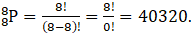9. How many words, with or without meaning can be made from the letters of the word MONDAY, assuming that no letter is repeated, if.
(i) 4 letters are used at a time,

(ii) All letters are used at a time,
(iii) all letters are used but first letter is a vowel?

Solution:

(i) Number of letters to be used =4

⇒ Number of permutations =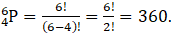(ii) Number of letters to be used = 6

⇒ Number of permutations =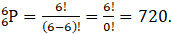(iii) Number of vowels in MONDAY = 2 (O and A)

⇒ Number of permutations in vowel =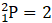Now, remaining places = 5

Remaining letters to be used =5

⇒ Number of permutations =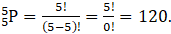Therefore, total number of permutations = 2 × 120 =240.

10. In how many of the distinct permutations of the letters in MISSISSIPPI do the four I’s not come together?

Solution:

Total number of letters in MISSISSIPPI =11

Letter Number of occurrence

 M 1 I 4 S 4 P 2

⇒ Number of permutations =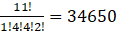We take that 4 I’s come together, and they are treated as 1 letter,

∴ Total number of letters=11 – 4 + 1 = 8

⇒ Number of permutations =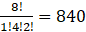Therefore, total number of permutations where four I’s don’t come together = 34650-840=33810

11. In how many ways can the letters of the word PERMUTATIONS be arranged if the

(ii) Vowels are all together,
(iii) There are always 4 letters between P and S?

Solution:

(i) Total number of letters in PERMUTATIONS =12

Only repeated letter is T; 2times

First and last letter of the word are fixed as P and S respectively.

Number of letters remaining =12 – 2 = 10

⇒ Number of permutations =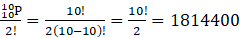(ii) Number of vowels in PERMUTATIONS = 5 (E, U, A, I, O)

Now, we consider all the vowels together as one.

Number of permutations of vowels = 120

Now total number of letters = 12 – 5 + 1= 8

⇒ Number of permutations =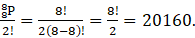Therefore, total number of permutations = 120 × 20160 = 2419200

(iii) Number of places are as 1 2 3 4 5 6 7 8 9 10 11 12

There should always be 4 letters between P and S.

Possible places of P and S are 1 and 6, 2and 7, 3 and 8, 4 and 9, 5 and 10, 6 and 11, 7 and 12

Possible ways =7,

Also, P and S can be interchanged,

No. of permutations =2 × 7 =14

Remaining 10 places can be filled with 10 remaining letters,

∴ No. of permutations =Therefore, total number of permutations = 14 × 1814400 =25401600.